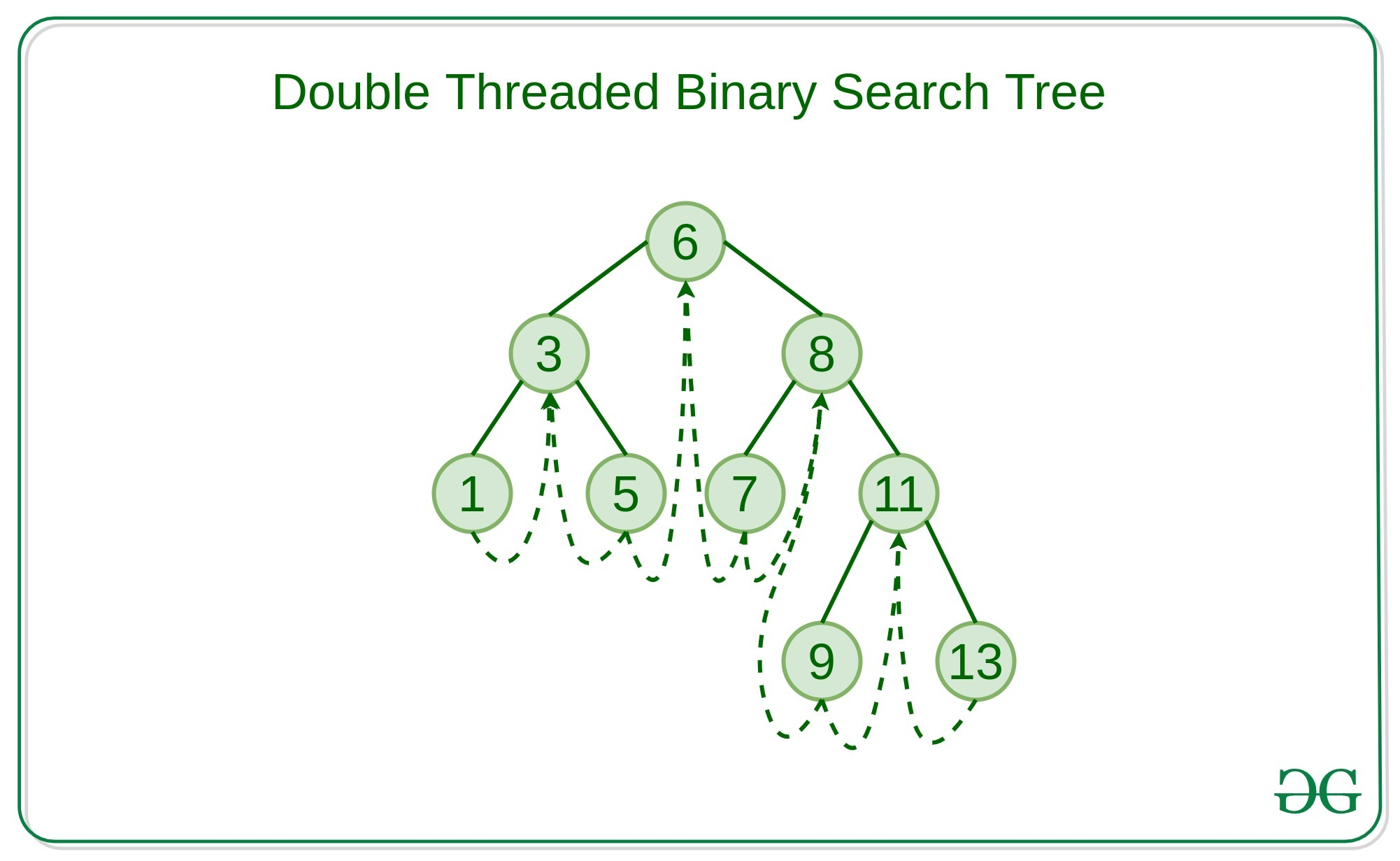Related Articles
Double Threaded Binary Search Tree
• Difficulty Level : Hard
• Last Updated : 19 Mar, 2021

Double Threaded Binary Search Tree: is a binary search tree in which the nodes are not every left NULL pointer points to its inorder predecessor and the right NULL pointer points to the inorder successor.
The threads are also useful for fast accessing the ancestors of a node.
Double Threaded Binary Search Tree is one of the most used types of Advanced data structures used in many real-time applications like places where there are recent insertion and traversal of all elements of the search tree.Creation algorithm for Double Threaded Binary Search Tree:

• In Double Threaded Binary search tree, there are five fields namely, data fields, left, right pointers, lbit, and rbit where lbit and rbit are boolean value stored to denote the right pointer points to an inorder successor or a new child node. Similarly, lbit denotes that the left pointer points to an inorder predecessor or a new child node.
• Base condition for the creation of the Double Threaded binary search tree is that the root node exists or not, If it doesn’t exist then create a new node and store it.
• Otherwise, compare the data of the current node to the new Data to be inserted, If the new data is less than the current data then traverse to the left child node. Otherwise, traverse to the right child node.
• If the left child or right child doesn’t exist then insert the node to its left and point its left and right child to the inorder predecessor and successor respectively.

Below is the implementation of the above approach:

## C++

 `// C++ implementation of the double``// threaded binary searighth tree` `#include ``using` `namespace` `std;` `// Class of the Node``class` `Node {``    ``int` `lbit, rbit;``    ``int` `value;``    ``Node *left, *right;` `public``:``    ``// Constructor of the``    ``// Node of the Tree``    ``Node()``    ``{``        ``lbit = rbit = 0;``        ``value = 0;``        ``left = right = NULL;``    ``}``    ``friend` `class` `DTBT;``};` `// Class of the Threaded``// Binary search tree``class` `DTBT {``    ``Node* root;` `public``:``    ``// Constructor of the``    ``// Threaded of the Binary``    ``// Search Tree``    ``DTBT()``    ``{``        ``root = ``new` `Node();` `        ``// Initalise the dummy node``        ``// to any random value of``        ``// your choice.``        ``root->value = 9999;` `        ``// Considering our whole``        ``// tree is at left of``        ``// dummy node``        ``root->rbit = 1;``        ``root->lbit = 0;` `        ``// Consider your whole tree``        ``// lies to the left of``        ``// this dummy node.``        ``root->left = root;``        ``root->right = root;``    ``}``    ``void` `create();``    ``void` `insert(``int` `value);``    ``void` `preorder();``    ``Node* preorderSuccessor(Node*);``    ``void` `inorder();``    ``Node* inorderSuccessor(Node*);``};` `// Function to create the Binary``// search tree``void` `DTBT::create()``{``    ``int` `n = 9;` `    ``// Insertion of the nodes``    ``this``->insert(6);``    ``this``->insert(3);``    ``this``->insert(1);``    ``this``->insert(5);``    ``this``->insert(8);``    ``this``->insert(7);``    ``this``->insert(11);``    ``this``->insert(9);``    ``this``->insert(13);``}` `// Function to insert the nodes``// into the threaded binary``// search tree``void` `DTBT::insert(``int` `data)``{``    ``// Condition to check if there``    ``// is no node in the binary tree``    ``if` `(root->left == root``        ``&& root->right == root) {` `        ``Node* p = ``new` `Node();``        ``p->value = data;``        ``p->left = root->left;``        ``p->lbit = root->lbit;``        ``p->rbit = 0;``        ``p->right = root->right;` `        ``// Inserting the node in the``        ``// left of the dummy node``        ``root->left = p;``        ``root->lbit = 1;``        ``return``;``    ``}` `    ``// New node``    ``Node* cur = ``new` `Node;``    ``cur = root->left;``    ``while` `(1) {``        ``// Condition to check if the``        ``// data to be inserted is``        ``// less than the current node``        ``if` `(cur->value < data) {``            ``Node* p = ``new` `Node();``            ``p->value = data;``            ``if` `(cur->rbit == 0) {``                ``p->right = cur->right;``                ``p->rbit = cur->rbit;``                ``p->lbit = 0;``                ``p->left = cur;` `                ``// Inserting the node``                ``// in the right``                ``cur->rbit = 1;``                ``cur->right = p;``                ``return``;``            ``}``            ``else``                ``cur = cur->right;``        ``}` `        ``// Otherwise insert the node``        ``// in the left of current node``        ``if` `(cur->value > data) {``            ``Node* p = ``new` `Node();``            ``p->value = data;``            ``if` `(cur->lbit == 0) {``                ``p->left = cur->left;``                ``p->lbit = cur->lbit;``                ``p->rbit = 0;` `                ``// Pointing the right child``                ``// to its inorder Successor``                ``p->right = cur;``                ``cur->lbit = 1;``                ``cur->left = p;``                ``return``;``            ``}``            ``else``                ``cur = cur->left;``        ``}``    ``}``}` `// In Threaded binary search tree``// the left pointer of every node``// points to its Inorder predecessor,``// whereas its right pointer points``// to the the Inorder Successor``void` `DTBT::preorder()``{``    ``Node* c = root->left;` `    ``// Loop to traverse the tree in``    ``// the preorder fashion``    ``while` `(c != root) {``        ``cout << ``" "` `<< c->value;``        ``c = preorderSuccessor(c);``    ``}``}` `// Function to find the preorder``// Successor of the node``Node* DTBT::preorderSuccessor(Node* c)``{``    ``if` `(c->lbit == 1) {``        ``return` `c->left;``    ``}``    ``while` `(c->rbit == 0) {``        ``c = c->right;``    ``}``    ``return` `c->right;``}` `// In Threaded binary search tree``// the left pointer of every node``// points to its Inorder predecessor``// whereas its right pointer points``// to the the Inorder Successor``void` `DTBT::inorder()``{``    ``Node* c;``    ``c = root->left;``    ``while` `(c->lbit == 1)``        ``c = c->left;` `    ``// Loop to traverse the tree``    ``while` `(c != root) {``        ``cout << ``" "` `<< c->value;``        ``c = inorderSuccessor(c);``    ``}``}` `// Function to find the inorder``// successor of the node``Node* DTBT::inorderSuccessor(Node* c)``{``    ``if` `(c->rbit == 0)``        ``return` `c->right;``    ``else``        ``c = c->right;``    ``while` `(c->lbit == 1) {``        ``c = c->left;``    ``}``    ``return` `c;``}` `// Driver Code``int` `main()``{``    ``DTBT t1;` `    ``// Creation of the Threaded``    ``// Binary search tree``    ``t1.create();` `    ``cout << ``"Inorder Travesal of DTBST\n"``;``    ``t1.inorder();` `    ``cout << ``"\nPreorder Travesal of DTBST\n"``;``    ``t1.preorder();``    ``return` `0;``}`
Output:
```Inorder Travesal of DTBST
1 3 5 6 7 8 9 11 13
Preorder Travesal of DTBST
6 3 1 5 8 7 11 9 13```

Attention reader! Don’t stop learning now. Get hold of all the important DSA concepts with the DSA Self Paced Course at a student-friendly price and become industry ready.  To complete your preparation from learning a language to DS Algo and many more,  please refer Complete Interview Preparation Course.

My Personal Notes arrow_drop_up